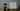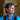# C# sorting - a subtle mistakeRecently I've learned a funny (ehm...) thing.

## The guilty

It isn't true that the inverse of a negative number is a positive number. Or, equally, that (x < 0) => (-x > 0).

You could say «Hey, -(-5) == 5». Yes, that's true. We can test it this way:

``````[Test]
public void TestInverse()
{
int x = -5;
int y = -x;
Assert.IsTrue(y > 0);
}``````

But what if we consider edge cases?

``````[Test]
public void TestInverse_EdgeCase()
{
int x = int.MinValue;
int y = -x;
Assert.IsTrue(y > 0);
}``````

It will fail. Miserably.

## The reason

The reason is simple: the sign occupies space. In fact, the range of int is -2,147,483,648 to 2,147,483,647. The inverse of -2,147,483,648 would cause overflow and returns the same value.

## The lesson

Why am I pointing at this?

Imagine you are implementing a `CompareTo(x, y)` method, you know, the usual one that returns 0 if the values are considered equal, -1 if x < y and 1 if x > y.

You could use this method to sort an array. Now you want to sort that array descending. What to do?

This edge case explains why it is a terrible idea to use `CompareTo(-x, -y)`. Results can be unexpected.

The best solution is to simply switch the parameters: `CompareTo(y, x)`.

## Conclusion

This example teaches us that we must know the basics of a language not only in terms of syntax but also in terms of inner handling. If we just used int without knowing how it is made, we would fall into this mistake without knowing why.### code4it

Ciao! I'm Davide Bellone, a .NET software developer! Let's keep in touch on Twitter!